# Sculpting in time

Do one thing and do it well.
Every story has a beginning and an end.

## Foundations of Modelica systemmodeler with Mathematica (1)

modelica Modeling Primer-1

FengChen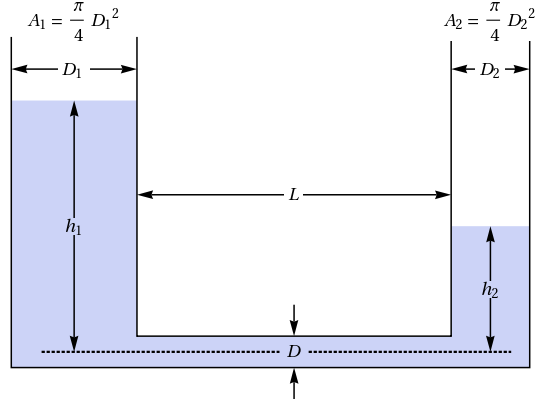## Why study Modelica

I have seen a book “Model Thinking” before, and this time it happens to be in the CoVid-2019 disease ravaging the world, by chance I saw the prediction model SEIR about epidemiology, I took this opportunity to start studying mathematical models, and decided to pick up the mathematical analysis, ordinary differential equations, partial differential equations, differential geometry and other courses I studied in college. I came across the language Modelica, and since I had been studying Wolfram Mathematica, I was quite fond of SystemModeler of the Wolfram product line, so I started to buy the home edition of SystemModeler and purchased a book “Introduction and Improvement of Modelica Multi-Domain Physical System Modeling”. I began my exploration of Modelica with the expectation of gaining a deeper understanding of mathematical model building, using mathematical models to predict the future development pattern of an event and gaining more hidden information in quantitative data analysis.

## Modeling - Hydraulic Systems

The language used is Mathematica.### The fluid is incompressible

First build a system of equations based on the Hagen-Poiseuille relationship: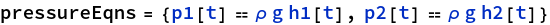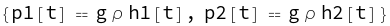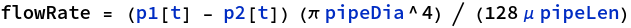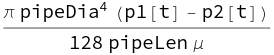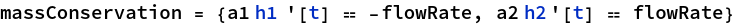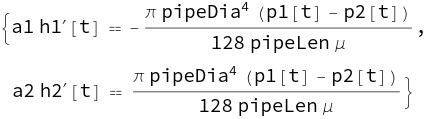Pre-set parameters: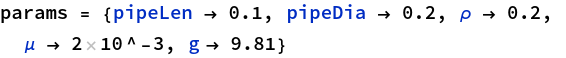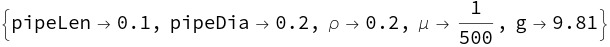Assuming that the cross-sectional area of the tubes on both sides is the same as 1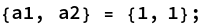Solve the above differential equation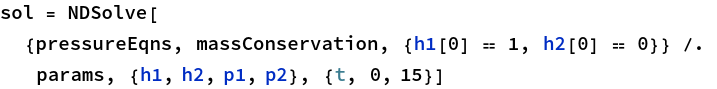Plots of height change of h1, h2 h1[t], h2[t]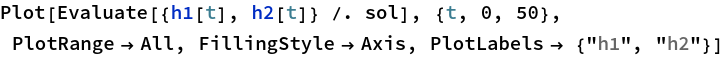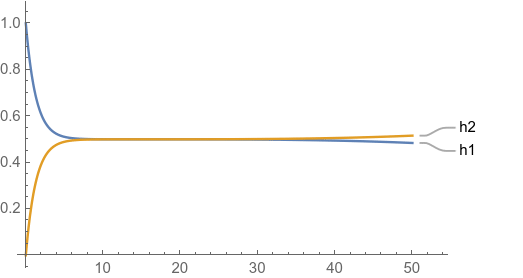The set of equations is shown below: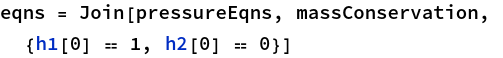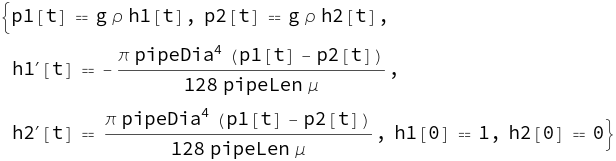Finally, create the SystemModeler model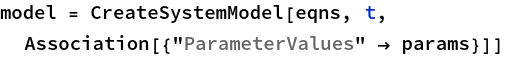``````model W8918fcdd72d8462a890fa97776822164
parameter Real g = 9.81;
parameter Real mu = 1 / 500;
parameter Real pipeDia = 0.2;
parameter Real pipeLen = 0.1;
parameter Real rho = 0.2;
Real h1;
Real h2;
Real p1;
Real p2;
initial equation
h1 = 1;
h2 = 0;
equation
p1 = g * rho * h1;
p2 = g * rho * h2;
der(h1) = (-1) / 128 * Modelica.Constants.pi * pipeDia ^ 4 * pipeLen ^ (-1) * mu ^ (-1) * (p1 + (-1) * p2);
der(h2) = 1 / 128 * Modelica.Constants.pi * pipeDia ^ 4 * pipeLen ^ (-1) * mu ^ (-1) * (p1 + (-1) * p2);
";
end W8918fcdd72d8462a890fa97776822164;
``````

Copy the Modelica source code to SystemModeler and run it to get the following graph of h1, h2 height change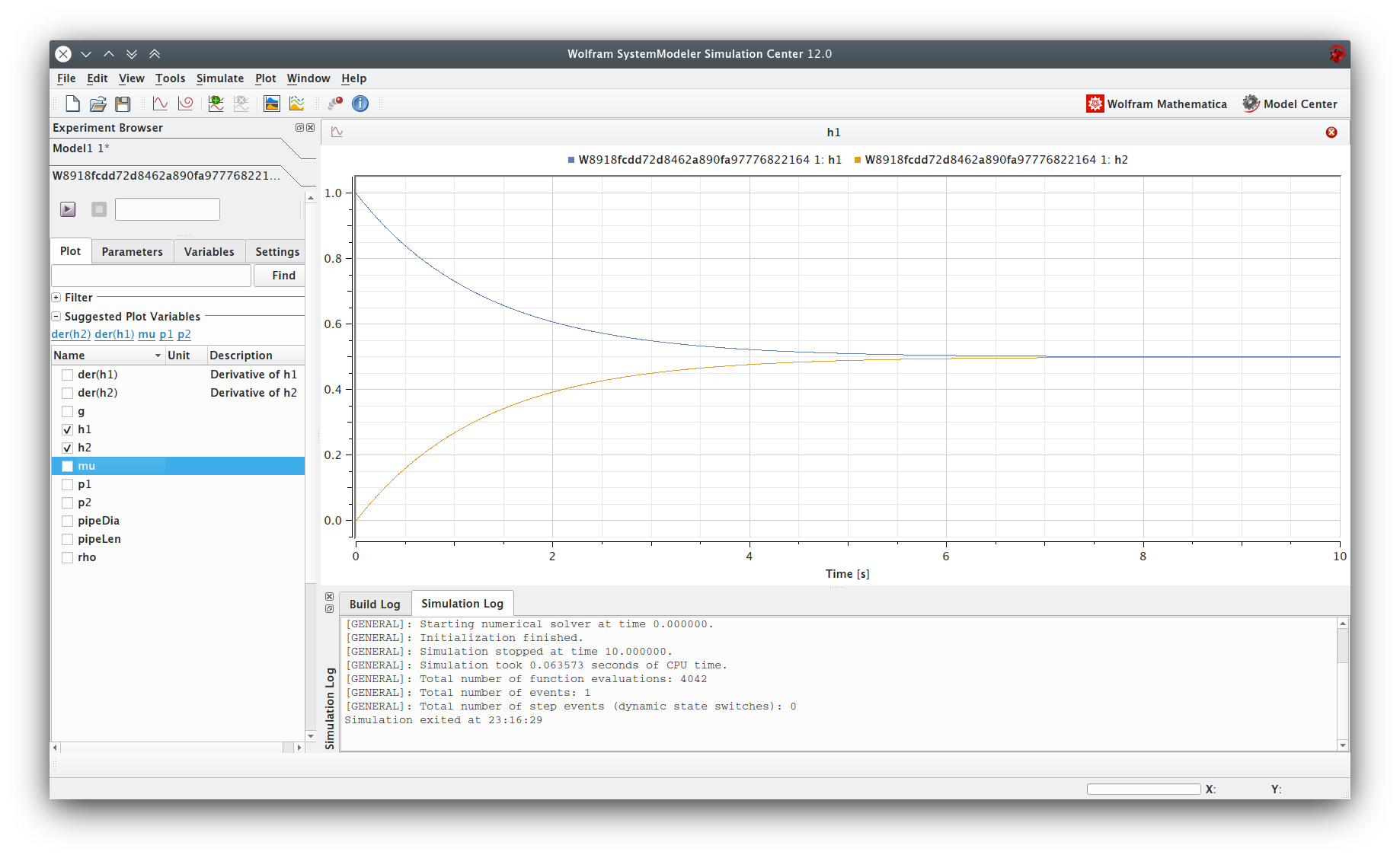## Summary

In the absence of Mathematica and SystemModeler combined use, for the first stage of rapid writing Modelica source code is a tricky thing, then check the Mathematica help documentation found that you can use CreateSystemModel to complete the automatic generation of code, the advantage is that you can completely The advantage is that you can create intuitive mathematical logic in Mathematica, and then automatically generate Modelica code by CreateSystemModel and put it into modeler for further analysis and processing.